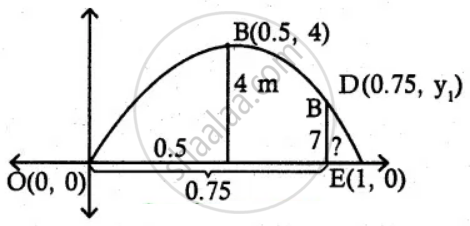Tamil Nadu Board of Secondary EducationHSC Arts Class 12th

# At a water fountain, water attains a maximum height of 4 m at horizontal distance of 0.5 m from its origin. If the path of water is a parabola, find the height of water at a horizontal - Mathematics

Sum

At a water fountain, water attains a maximum height of 4 m at horizontal distance of 0.5 m from its origin. If the path of water is a parabola, find the height of water at a horizontal distance of 0.75 m from the point of origin.

#### SolutionLet the equation of parabola be

(x – h)2 = – 4a(y – k)

Here vertex B(0.5, 4)

∴ Equation of parabola

(x – 0.5)2 = – 4a(y – 4)

Parabola passes through origin (0, 0)

(0 – 0.5)2 = – 4a(0 – 4)

(- 1/2)^2 = 16a

∴ 1/4 = 16a

⇒ a =1/64

∴ Equation of parabola

(x – 0.5)2 = - 4 (1/64)(y - 4)

This Parabola passes again through D(0.75, y1)

∴ (0.75 – 0.5)2 = - 1/16 (y_1 - 4)

(0.25)= - 1/16 (y_1 - 4)

(1/4)^2 = - 1/16 (y_1 - 4)

1/16 = - 1/16 (y_1 - 4)

1 = – y1 + 4

y1 = 3

Height of water at a horizontal distance of 0.75 m is 3 m.

Concept: Real Life Applications of Conics
Is there an error in this question or solution?
Chapter 5: Two Dimensional Analytical Geometry-II - Exercise 5.5 [Page 214]

#### APPEARS IN

Tamil Nadu Board Samacheer Kalvi Class 12th Mathematics Volume 1 and 2 Answers Guide
Chapter 5 Two Dimensional Analytical Geometry-II
Exercise 5.5 | Q 3 | Page 214
Share